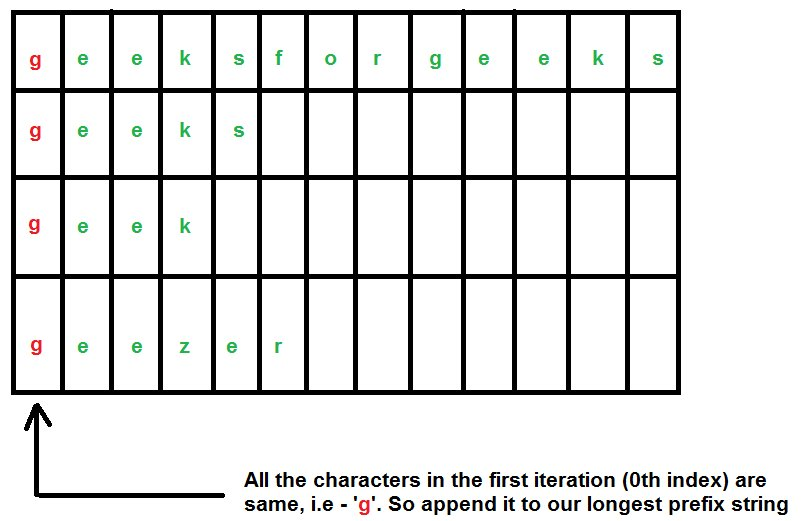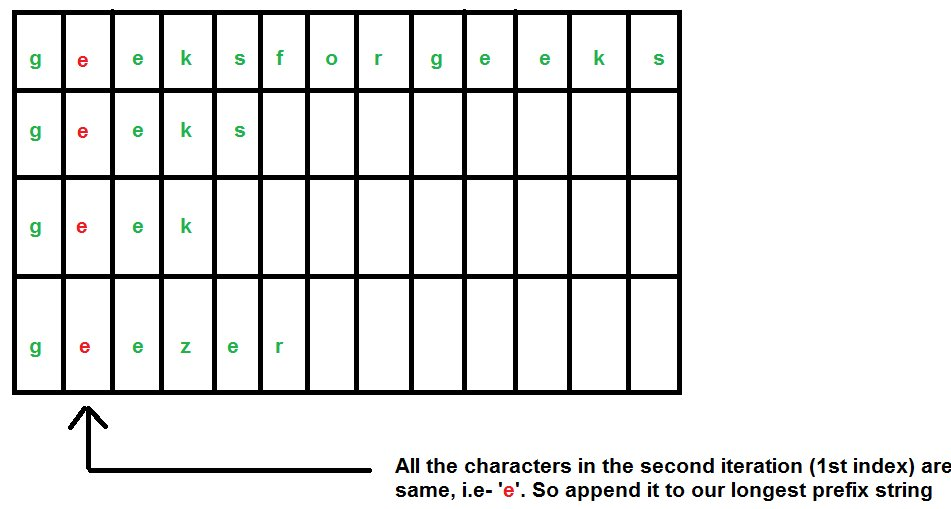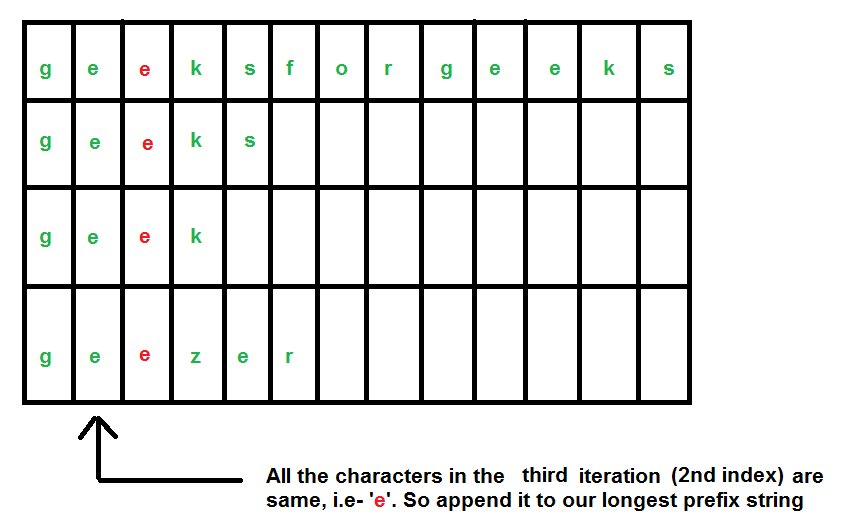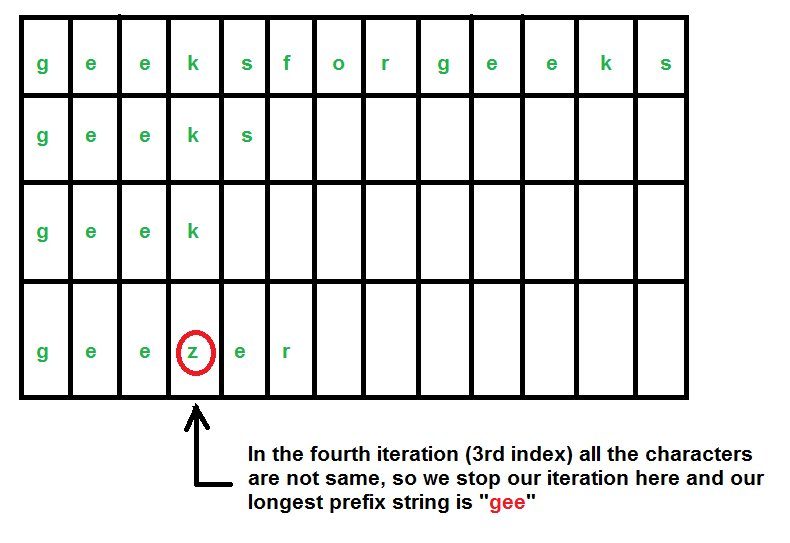Skip to content
Related Articles
Longest Common Prefix using Character by Character Matching
• Difficulty Level : Easy
• Last Updated : 08 Apr, 2021

Given a set of strings, find the longest common prefix.

```Input  : {“geeksforgeeks”, “geeks”, “geek”, “geezer”}
Output : "gee"

Input  : {"apple", "ape", "april"}
Output : "ap"```

We have discussed word by word matching algorithm in previous post.
In this algorithm, instead of going through the strings one by one, we will go through the characters one by one.
We consider our strings as – “geeksforgeeks”, “geeks”, “geek”, “geezer”.Below is the implementation of this approach.

## C++

 `//  A C++ Program to find the longest common prefix``#include``using` `namespace` `std;` `// A Function to find the string having the minimum``// length and returns that length``int` `findMinLength(string arr[], ``int` `n)``{``    ``int` `min = arr.length();` `    ``for` `(``int` `i=1; i

## Java

 `// A Java Program to find the longest common prefix``class` `GFG``{` `    ``// A Function to find the string having the minimum``    ``// length and returns that length``    ``static` `int` `findMinLength(String arr[], ``int` `n)``    ``{``        ``int` `min = arr[``0``].length();` `        ``for` `(``int` `i = ``1``; i < n; i++)``        ``{``            ``if` `(arr[i].length() < min)``            ``{``                ``min = arr[i].length();``            ``}``        ``}` `        ``return` `(min);``    ``}` `    ``// A Function that returns the longest common prefix``    ``// from the array of strings``    ``static` `String commonPrefix(String arr[], ``int` `n)``    ``{``        ``int` `minlen = findMinLength(arr, n);` `        ``String result = ``""``; ``// Our resultant string``        ``char` `current; ``// The current character` `        ``for` `(``int` `i = ``0``; i < minlen; i++)``        ``{``            ``// Current character (must be same``            ``// in all strings to be a part of``            ``// result)``            ``current = arr[``0``].charAt(i);` `            ``for` `(``int` `j = ``1``; j < n; j++)``            ``{``                ``if` `(arr[j].charAt(i) != current)``                ``{``                    ``return` `result;``                ``}``            ``}` `            ``// Append to result``            ``result += (current);``        ``}` `        ``return` `(result);``    ``}` `    ``// Driver program to test above function``    ``public` `static` `void` `main(String[] args)``    ``{``        ``String arr[] = {``"geeksforgeeks"``, ``"geeks"``,``            ``"geek"``, ``"geezer"``};``        ``int` `n = arr.length;` `        ``String ans = commonPrefix(arr, n);` `        ``if` `(ans.length() > ``0``) {``            ``System.out.println(``"The longest common prefix is "``                    ``+ ans);``        ``} ``else` `{``            ``System.out.println(``"There is no common prefix"``);``        ``}``    ``}``}` `// This code contributed by Rajput-Ji`

## Python 3

 `# Python 3 Program to find the longest common prefix`` ` `# A Function to find the string having the minimum``# length and returns that length``def` `findMinLength(arr, n):` `    ``min` `=` `len``(arr[``0``])`` ` `    ``for` `i ``in` `range``(``1``,n):``        ``if` `(``len``(arr[i])< ``min``):``            ``min` `=` `len``(arr[i])`` ` `    ``return``(``min``)`` ` `# A Function that returns the longest common prefix``# from the array of strings``def` `commonPrefix(arr, n):` `    ``minlen ``=` `findMinLength(arr, n)``    ``result ``=``""``    ``for` `i ``in` `range``(minlen):``    ` `        ``# Current character (must be same``        ``# in all strings to be a part of``        ``# result)``        ``current ``=` `arr[``0``][i]`` ` `        ``for` `j ``in` `range``(``1``,n):``            ``if` `(arr[j][i] !``=` `current):``                ``return` `result`` ` `        ``# Append to result``        ``result ``=` `result``+``current`` ` `    ``return` `(result)`` ` `# Driver program to test above function``if` `__name__ ``=``=` `"__main__"``:``    ` `    ``arr ``=` `[``"geeksforgeeks"``, ``"geeks"``,``                    ``"geek"``, ``"geezer"``]``    ``n ``=` `len``(arr)`` ` `    ``ans ``=` `commonPrefix (arr, n)`` ` `    ``if` `(``len``(ans)):``        ``print``(``"The longest common prefix is "``,ans)``    ``else``:``        ``print``(``"There is no common prefix"``)`

## C#

 `// A C# Program to find the longest common prefix``using` `System;``    ` `class` `GFG``{` `    ``// A Function to find the string having the minimum``    ``// length and returns that length``    ``static` `int` `findMinLength(String []arr, ``int` `n)``    ``{``        ``int` `min = arr.Length;` `        ``for` `(``int` `i = 1; i < n; i++)``        ``{``            ``if` `(arr[i].Length < min)``            ``{``                ``min = arr[i].Length;``            ``}``        ``}` `        ``return` `(min);``    ``}` `    ``// A Function that returns the longest common prefix``    ``// from the array of strings``    ``static` `String commonPrefix(String []arr, ``int` `n)``    ``{``        ``int` `minlen = findMinLength(arr, n);` `        ``String result = ``""``; ``// Our resultant string``        ``char` `current; ``// The current character` `        ``for` `(``int` `i = 0; i < minlen; i++)``        ``{``            ``// Current character (must be same``            ``// in all strings to be a part of``            ``// result)``            ``current = arr[i];` `            ``for` `(``int` `j = 1; j < n; j++)``            ``{``                ``if` `(arr[j][i] != current)``                ``{``                    ``return` `result;``                ``}``            ``}` `            ``// Append to result``            ``result += (current);``        ``}` `        ``return` `(result);``    ``}` `    ``// Driver code``    ``public` `static` `void` `Main(String[] args)``    ``{``        ``String []arr = {``"geeksforgeeks"``, ``"geeks"``,``            ``"geek"``, ``"geezer"``};``        ``int` `n = arr.Length;` `        ``String ans = commonPrefix(arr, n);` `        ``if` `(ans.Length > 0)``        ``{``            ``Console.WriteLine(``"The longest common prefix is "``                    ``+ ans);``        ``}``        ``else``        ``{``            ``Console.WriteLine(``"There is no common prefix"``);``        ``}``    ``}``}` `/* This code contributed by PrinciRaj1992 */`

## Javascript

 ``

Output :

`The longest common prefix is  gee`

How is this algorithm better than the “Word by Word Matching” algorithm ?-
In Set 1 we discussed about the “Word by Word Matching” Algorithm.
Suppose you have the input strings as- “geeksforgeeks”, “geeks”, “geek”, “geezer”, “x”.
Now there is no common prefix string of the above strings. By the “Word by Word Matching” algorithm discussed in Set 1, we come to the conclusion that there is no common prefix string by traversing all the strings. But if we use this algorithm, then in the first iteration itself we will come to know that there is no common prefix string, as we don’t go further to look for the second character of each strings.
This algorithm has a huge advantage when there are too many strings.
Time Complexity : Since we are iterating through all the characters of all the strings, so we can say that the time complexity is O(N M) where,

```N = Number of strings
M = Length of the largest string string ```

Auxiliary Space : To store the longest prefix string we are allocating space which is O(M).
This article is contributed by Rachit Belwariar. If you like GeeksforGeeks and would like to contribute, you can also write an article and mail your article to contribute@geeksforgeeks.org. See your article appearing on the GeeksforGeeks main page and help other Geeks.
Please write comments if you find anything incorrect, or you want to share more information about the topic discussed above

Attention reader! Don’t stop learning now. Get hold of all the important DSA concepts with the DSA Self Paced Course at a student-friendly price and become industry ready.  To complete your preparation from learning a language to DS Algo and many more,  please refer Complete Interview Preparation Course.

In case you wish to attend live classes with industry experts, please refer DSA Live Classes

My Personal Notes arrow_drop_up